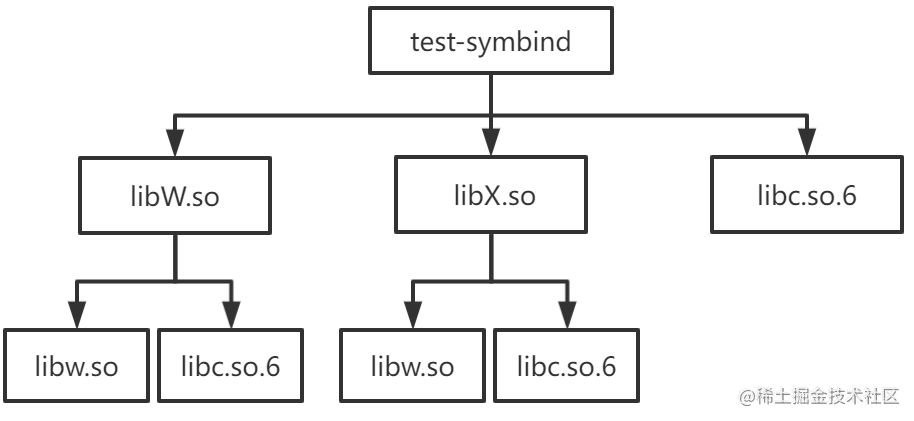# 深入淺出 Sanitizer Interceptor 機制

## Sanitizer 簡介

Sanitizer 是由 Google 開源的一系列動態程式碼分析工具，從 Clang 3.1 和 GCC 4.8 開始被整合在 Clang 和 GCC 中，能夠幫助程式設計師快速準確地在執行時定位程式中的記憶體錯誤和多執行緒錯誤。Sanitizer 工具集包括：

• LeakSanitizer (LSan)：用於檢測記憶體洩漏
• UndefinedBehaviorSanitizer (UBSsan)：用於檢測未定義行為
• MemorySanitizer (MSan)：用於檢測未初始化記憶體的訪問

• ASan 編譯時會在每一處記憶體讀寫語句之前插入程式碼，根據每一次訪問的記憶體所對應的影子記憶體 ( shadow memory，就是使用額外的記憶體來記錄常規的記憶體狀態）的狀態來檢測本次記憶體訪問是否合法。還會在棧變數和全域性變數附近申請額外記憶體作為危險區，用於檢測記憶體溢位。
• ASan 執行時庫會替換 malloc/free, operator new/delete 等記憶體分配函式的實現，這樣應用程式的記憶體分配都由 ASan 實現的記憶體分配器負責。ASan 記憶體分配器會在它分配的堆記憶體附近申請額外記憶體用於檢測堆記憶體溢位，還會將被釋放的記憶體優先放在隔離區 (quarantine) 用於檢測像 heap-use-after-free 這樣的堆記憶體錯誤。

## Symbol interposition

1. 一個最簡單的方式就是在我們的應用程式中定義一個同名的 malloc 函式
2. 還有一種方式就是將我們的 malloc 函式實現在 libmymalloc.so 中，然後在執行我們的應用程式之前設定環境變數 `LD_PRELOAD=/path/to/libmymalloc.so`

When resolving symbolic references, the dynamic linker examines the symbol tables with a breadth-first search. That is, it first looks at the symbol table of the executable program itself, then at the symbol tables of the `DT_NEEDED` entries (in order), and then at the second level `DT_NEEDED` entries, and so on.

``````\$ cat main.c
extern int W(), X();

int main() { return (W() + X()); }

\$ cat W.c
extern int b();

int a() { return (1); }
int W() { return (a() - b()); }

\$ cat w.c
int b() { return (2); }

\$ cat X.c
extern int b();

int a() { return (3); }
int X() { return (a() - b()); }

\$ cat x.c
int b() { return (4); }

\$ gcc -o libw.so -shared w.c
\$ gcc -o libW.so -shared W.c -L. -lw -Wl,-rpath=.
\$ gcc -o libx.so -shared x.c
\$ gcc -o libX.so -shared X.c -L. -lx -Wl,-rpath=.
\$ gcc -o test-symbind main.c -L. -lW -lX -Wl,-rpath=.

````````````\$ LD_DEBUG="symbols:bindings" ./test-symbind
1884890:        symbol=a;  lookup in file=./test-symbind 
1884890:        symbol=a;  lookup in file=./libW.so 
1884890:        binding file ./libW.so  to ./libW.so : normal symbol `a'
1884890:        symbol=b;  lookup in file=./test-symbind 
1884890:        symbol=b;  lookup in file=./libW.so 
1884890:        symbol=b;  lookup in file=./libX.so 
1884890:        symbol=b;  lookup in file=/lib/x86_64-linux-gnu/libc.so.6 
1884890:        symbol=b;  lookup in file=./libw.so 
1884890:        binding file ./libW.so  to ./libw.so : normal symbol `b'
1884890:        symbol=a;  lookup in file=./test-symbind 
1884890:        symbol=a;  lookup in file=./libW.so 
1884890:        binding file ./libX.so  to ./libW.so : normal symbol `a'
1884890:        symbol=b;  lookup in file=./test-symbind 
1884890:        symbol=b;  lookup in file=./libW.so 
1884890:        symbol=b;  lookup in file=./libX.so 
1884890:        symbol=b;  lookup in file=/lib/x86_64-linux-gnu/libc.so.6 
1884890:        symbol=b;  lookup in file=./libw.so 
1884890:        binding file ./libX.so  to ./libw.so : normal symbol `b'

``````
• 函式 a 在 libW.solibX.so 中都有一份定義，但因為是按照 test-symbind, libW.so, libX.so, libc.so.6, libw.so, libx.so 的順序查詢符號定義的，所以最終所有對函式 a 的引用都繫結到 libW.so 中函式 a 的實現
• 函式 b 在 libw.solibx.so 中都有一份定義，但因為是按照 test-symbind, libW.so, libX.so, libc.so.6, libw.so, libx.so 的順序查詢符號定義的，所以最終所有對函式 b 的引用都繫結到 libw.so 中函式 b 的實現

• 方式一：在我們的應用程式中定義一個同名的 malloc 函式。動態連結器在查詢符號時 executable 的順序在 libc.so.6 之前，因此所有對 malloc 的引用都會繫結到 executable 中 malloc 的實現。
• 方式二：將我們的 malloc 函式實現在 libmymalloc.so 中，然後在執行我們的應用程式之前設定環境變數 LD_PRELOAD=/path/to/libmymalloc.so。動態連結器在查詢符號時 libmymalloc.so 的順序在 libc.so.6 之前，因此所有對 malloc 的引用都會繫結到 libmymalloc.so 中 malloc 的實現。

``````// test.cpp
#include <iostream>
int main() {
}

``````

``````\$ objdump -p test-gcc-asan | grep NEEDED
NEEDED               libasan.so.5
NEEDED               libstdc++.so.6
NEEDED               libm.so.6
NEEDED               libgcc_s.so.1
NEEDED               libc.so.6

``````

``````\$ LD_DEBUG="bindings" ./test-gcc-asan
3309213:        binding file /lib/x86_64-linux-gnu/libc.so.6  to /usr/lib/x86_64-linux-gnu/libasan.so.5 : normal symbol `malloc' [GLIBC_2.2.5]
3309213:        binding file /lib64/ld-linux-x86-64.so.2  to /usr/lib/x86_64-linux-gnu/libasan.so.5 : normal symbol `malloc' [GLIBC_2.2.5]
3309213:        binding file /usr/lib/x86_64-linux-gnu/libstdc++.so.6  to /usr/lib/x86_64-linux-gnu/libasan.so.5 : normal symbol `malloc' [GLIBC_2.2.5]

``````

## Sanitizer interceptor

Sanitizer interceptor 存在一個測試檔案interception_linux_test.cpp

``````#include "interception/interception.h"
#include "gtest/gtest.h"

static int InterceptorFunctionCalled;

DECLARE_REAL(int, isdigit, int);

INTERCEPTOR(int, isdigit, int d) {
++InterceptorFunctionCalled;
return d >= '0' && d <= '9';
}

namespace __interception {

TEST(Interception, Basic) {
EXPECT_TRUE(INTERCEPT_FUNCTION(isdigit));

// After interception, the counter should be incremented.
InterceptorFunctionCalled = 0;
EXPECT_NE(0, isdigit('1'));
EXPECT_EQ(1, InterceptorFunctionCalled);
EXPECT_EQ(0, isdigit('a'));
EXPECT_EQ(2, InterceptorFunctionCalled);

// Calling the REAL function should not affect the counter.
InterceptorFunctionCalled = 0;
EXPECT_NE(0, REAL(isdigit)('1'));
EXPECT_EQ(0, REAL(isdigit)('a'));
EXPECT_EQ(0, InterceptorFunctionCalled);
}

}  // namespace __interception

``````

• `INTERCEPTOR(int, isdigit, int d) { ... }` 用於將函式 `isdigit` 的實現替換為 { ... } 的實現
• 在程式碼中呼叫 `isdigit` 之前，需要先呼叫 `INTERCEPT_FUNCTION(isdigit)`。如果 `INTERCEPT_FUNCTION(isdigit)` 返回為 true，則說明成功替換了將 libc 中 `isdigit` 函式的實現。
• `REAL(isdigit)('1')` 用於呼叫真正的 `isdigit` 實現，不過在呼叫 `REAL(isdigit)('1')` 之前需要先 `DECLARE_REAL(int, isdigit, int)`

``````INTERCEPTOR(int, isdigit, int d) {
++InterceptorFunctionCalled;
return d >= '0' && d <= '9';
}

INTERCEPT_FUNCTION(isdigit);

DECLARE_REAL(int, isdigit, int);
REAL(isdigit)('1');

``````
• 我們首先看下 INTERCEPTOR 巨集做了哪些事情

• 首先在 __interception namespace 中定義了一個函式指標 real_isdigit，該函式指標實際上在 INTERCEPT_FUNCTION 巨集中會被設定為指向真正的 `isdigit` 函式地址。
• 然後將 `isdigit` 函式設定為弱符號 (weak)，並且將 `isdigit` 設定成 `__interceptor_isdigit` 的別名 (alias)。
• 最後將我們自己版本的 `isdigit` 函式邏輯實現在 `__interceptor_isdigit` 函式中

``````extern "C" int __interceptor_isdigit(int d);
extern "C" int isdigit(int d) {
fprintf(stderr, "my_isdigit_interceptor\n");
return __interceptor_isdigit(d);
}

``````

• 接著我們看下 INTERCEPT_FUNCTION 巨集做了哪些事情

• INTERCEPT_FUNCTION 巨集展開後就是對 __interception::InterceptFunction 函式的呼叫。`InterceptFunction`函式定義
``````namespace __interception {
// If the lookup using RTLD_NEXT failed, the sanitizer 執行時庫 is
// later in the library search order than the DSO that we are trying to
// intercept, which means that we cannot intercept this function. We still
// want the address of the real definition, though, so look it up using
// RTLD_DEFAULT.

// In case `name' is not loaded, dlsym ends up finding the actual wrapper.
// We don't want to intercept the wrapper and have it point to itself.
}
}

bool InterceptFunction(const char *name, uptr *ptr_to_real, uptr func,
uptr wrapper) {
return addr && (func == wrapper);
}
}  // namespace __interception

``````

• dlsym 的第一個引數為 RTLD_DEFAULT 時，查詢名為 name 的函式地址的順序就是前面提到的 executable, preload0.so, preload1.so needed0.so, needed1.so, needed2.so, needed0_of_needed0.so, needed1_of_needed0.so, ... 這個順序。

• dlsym 的第一個引數為 RTLD_NEXT 時，則是以當前 object 後面動態庫為起點去查詢名為 name 的函式的地址

• 最後我們看下 DECLARE_REAL 巨集 和 REAL 巨集做了哪些事情

DECLARE_REAL 展開後就是聲明瞭在 __interception namespace 中存在一個指向被替換函式真正實現的函式指標，REAL 巨集就是通過這個函式指標來呼叫被替換函式的真正實現。

## 總結

ASan 基於 sanitizer interceptor 機制替換了 malloc/free 這類的記憶體分配/釋放函式，使得所有的記憶體分配和釋放都由 ASan 實現的記憶體分配器負責，這樣 ASan 就能很容易檢測到 heap-use-after-free，double-free 這樣的堆記憶體錯誤。

## 直播預告